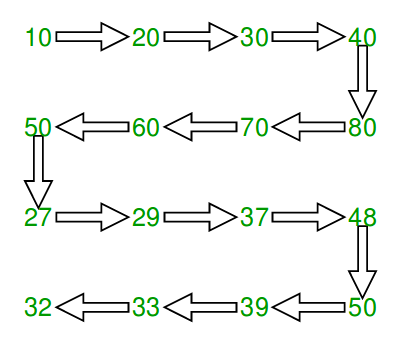# Java Program to Print matrix in snake pattern

• Last Updated : 11 Jan, 2022

Given an n x n matrix .In the given matrix, you have to print the elements of the matrix in the snake pattern.

Examples :

```Input :mat[][] = { {10, 20, 30, 40},
{15, 25, 35, 45},
{27, 29, 37, 48},
{32, 33, 39, 50}};

Output : 10 20 30 40 45 35 25 15 27 29
37 48 50 39 33 32

Input :mat[][] = { {1, 2, 3},
{4, 5, 6},
{7, 8, 9}};
Output : 1 2 3 6 5 4 7 8 9```We traverse all rows. For every row, we check if it is even or odd. If even, we print from left to right else print from right to left.

## Java

 `// Java program to print matrix in snake order``import` `java.util.*;``class` `GFG``{``    ``static` `void` `print(``int` `[][] mat)``    ``{``        ``// Traverse through all rows``        ``for` `(``int` `i = ``0``; i < mat.length; i++)``        ``{`` ` `            ``// If current row is even, print from``            ``// left to right``            ``if` `(i % ``2` `== ``0``)``            ``{``                ``for` `(``int` `j = ``0``; j < mat[``0``].length; j++)``                    ``System.out.print(mat[i][j] +``" "``);`` ` ` ` `            ``// If current row is odd, print from``            ``// right to left``            ``}``            ``else``            ``{``                ``for` `(``int` `j = mat[``0``].length - ``1``; j >= ``0``; j--)``                    ``System.out.print(mat[i][j] +``" "``);``            ``}``        ``}``    ``}`` ` `    ``// Driver code``    ``public` `static` `void` `main(String[] args)``    ``{``        ``int` `mat[][] = ``new` `int``[][]``        ``{``            ``{ ``10``, ``20``, ``30``, ``40` `},``            ``{ ``15``, ``25``, ``35``, ``45` `},``            ``{ ``27``, ``29``, ``37``, ``48` `},``            ``{ ``32``, ``33``, ``39``, ``50` `}``        ``};`` ` `        ``print(mat);``    ``}``}``/* This code is contributed by Mr. Somesh Awasthi */`

Output :

`10 20 30 40 45 35 25 15 27 29 37 48 50 39 33 32 `

Please refer complete article on Print matrix in snake pattern for more details!

My Personal Notes arrow_drop_up